# 一、简介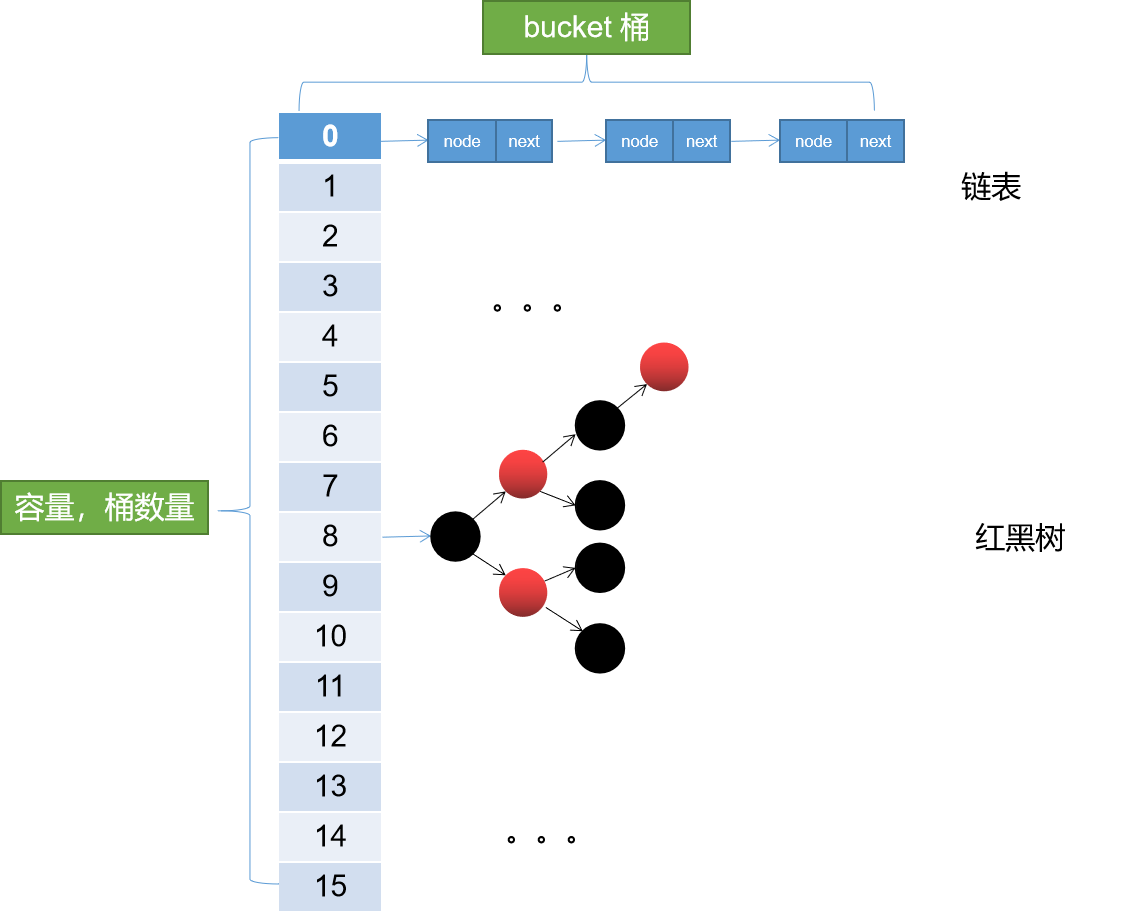呃呵，和HashMap的结构是一样的，没错在数据结构层面，ConcurrentHashMap和HashMap是完全一样的。有了这个基础继续往下看。

# 二、历史版本

ConcurrentHashMap的历史版本大致分界线在JDK8。也就是可以分为JDK8和JDK8以前版本。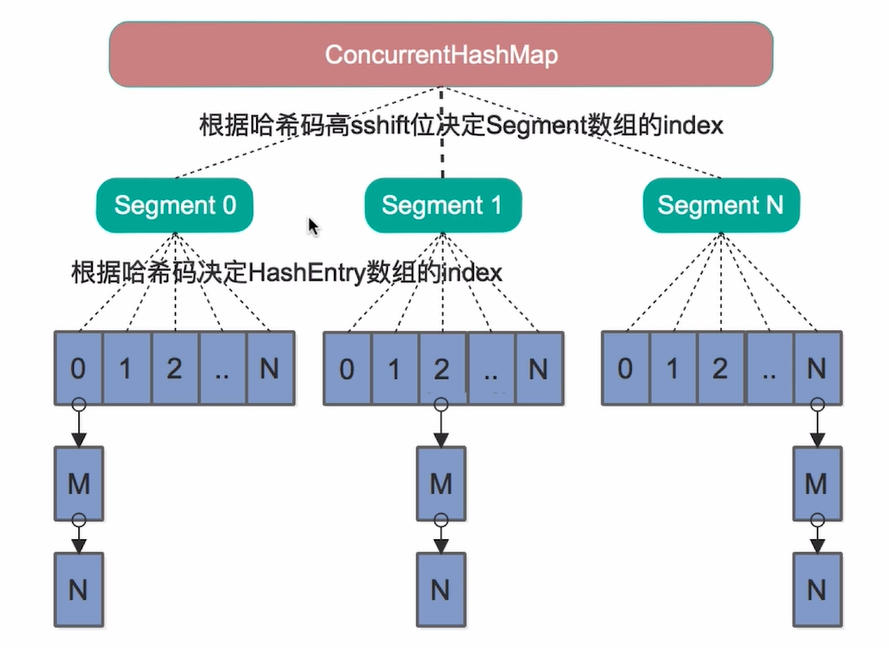从上图我们不难看出其在数据结构方面的差别。

JDK7中为了提高并发性能采用了这种分段的设计。所以在JDK7中ConcurrentHashMap采用的是分段锁，也就是在每个Segment上加ReentrantLock实现的线程安全线。关于ReetrantLock后面有时间会介绍，大致来说ReetrantLoack是比Synchronized更细粒度的一种锁。使用得当的话其性能要比Synchronized表现要好，但是如果实现不得当容易造成死锁。

JDK8中ConcurrentHashMap采用的是CAS+Synchronized锁并且锁粒度是每一个桶。简单来说JDK7中锁的粒度是Segment，JDK8锁粒度细化到了桶级别。可想而知锁粒度是大大提到了。辅之以代码的优化，JDK8中的ConcurrentHashMap在性能上的表现非常优秀。

# 三、基础知识

## 3.1、常量

//正在扩容，对应fwd类型的节点的hash
static final int MOVED     = -1; // hash for forwarding nodes
//当前数组
transient volatile Node<K,V>[] table;
//扩容时用到的，扩容后的数组。
private transient volatile Node<K,V>[] nextTable;
//1，大于零，表示size * 0.75。
//2，等于-1，表示正在初始化。
//3，-(n + 1)，表示正在执行扩容的线程其只表示基数，而不是真正的数量，需要计算得出的哦
private transient volatile int sizeCtl;

## 3.2、Unsafe类方法

 1     @SuppressWarnings("unchecked")  //transient volatile Node<K,V>[] table; tab变量确实是volatile
2     static final <K,V> Node<K,V> tabAt(Node<K,V>[] tab, int i) {//获取table中索引 i 处的元素。
3         return (Node<K,V>)U.getObjectVolatile(tab, ((long)i << ASHIFT) + ABASE);//如果tab是volatile变量，则该方法保证其可见性。
4     }
5
6     static final <K,V> boolean casTabAt(Node<K,V>[] tab, int i,//通过CAS设置table索引为 i 处的元素。
7                                         Node<K,V> c, Node<K,V> v) {
8         return U.compareAndSwapObject(tab, ((long)i << ASHIFT) + ABASE, c, v);
9     }
10             //transient volatile Node<K,V>[] table; tab变量确实是volatile
11     static final <K,V> void setTabAt(Node<K,V>[] tab, int i, Node<K,V> v) {//修改table 索引 i 处的元素。
12         U.putObjectVolatile(tab, ((long)i << ASHIFT) + ABASE, v);//如果tab是volatile变量，则该方法保证其可见性。
13     }

tabAt和setTabAt最终调用的两个方法分别是 U.getObjectVolatile()和U.putObjectVolatile 顾名思义其是通过volatile保证的tab的可见性（Volatile只保证可见性不保证原子性哦）。前提是tab变量是Volatile修饰的变量。我们通过调用栈，最红可以看到其实tab就是ConcurrentHashMap中的table。而这个变量是这么定义的。

transient volatile Node<K,V>[] table;

casTabAt方法，这个就是CAS方法了。

CAS：Compare and Swap三个单词的缩写，即：比较交换的意思。CAS在Java中又称之为乐观锁即我们总认为是没有锁的。

while(true){
CAS();
}

# 四、put过程源码

 1 public V put(K key, V value) {
2     return putVal(key, value, false);
3 }
4
5 /** Implementation for put and putIfAbsent */
6 final V putVal(K key, V value, boolean onlyIfAbsent) {
7     if (key == null || value == null) throw new NullPointerException();
9     int binCount = 0;
10     for (Node<K,V>[] tab = table;;) {//迭代桶数组，自旋
11         Node<K,V> f; int n, i, fh;
12         if (tab == null || (n = tab.length) == 0)//懒加载。如果为空，则进行初始化
13             tab = initTable();//初始化桶数组
14         //(n - 1) & hash)计算下标，取值，为空即无hash碰撞
15         else if ((f = tabAt(tab, i = (n - 1) & hash)) == null) {
16             if (casTabAt(tab, i, null,
17                          new Node<K,V>(hash, key, value, null)))//通过cas插入新值
18                 break;                   // no lock when adding to empty bin
19         }
20         //判断是否正在扩容。如果正在扩容，当前线程帮助进行扩容。
21         //每个线程只能同时负责一个桶上的数据迁移，并且不影响其它桶的put和get操作。
22         //（很牛逼的思路，能这么做建立在更细粒度的锁基础上）
23         else if ((fh = f.hash) == MOVED)
24             tab = helpTransfer(tab, f);
25         else {//put5,存在hash碰撞
26             V oldVal = null;
27             //此处，f在上面已经被赋值，f为当前下标桶的首元素。对链表来说是链表头对红黑树来说是红黑树的头元素。
28             synchronized (f) {
29                 //再次检查当前节点是否有变化，有变化进入下一轮自旋
30                 //为什么再次检查？因为不能保证，当前线程到这里，有没有其他线程对该节点进行修改
31                 if (tabAt(tab, i) == f) {
32                     if (fh >= 0) {//当前桶为链表
33                         binCount = 1;
34                         for (Node<K,V> e = f;; ++binCount) {//迭代链表节点
35                             K ek;
36                             if (e.hash == hash &&//key相同，覆盖（onlyIfAbsent有什么用？）
37                                 ((ek = e.key) == key ||
38                                  (ek != null && key.equals(ek)))) {
39                                 oldVal = e.val;
40                                 if (!onlyIfAbsent)
41                                     e.val = value;
42                                 break;
43                             }
44                             Node<K,V> pred = e;
45                             //找到链表尾部，插入新节点。（什么这里不用CAS？因为这在同步代码块里面）
46                             if ((e = e.next) == null) {
47                                 pred.next = new Node<K,V>(hash, key,
48                                                           value, null);
49                                 break;
50                             }
51                         }
52                     }
53                     else if (f instanceof TreeBin) {//当前桶为红黑树
54                         Node<K,V> p;
55                         binCount = 2;
56                         if ((p = ((TreeBin<K,V>)f).putTreeVal(hash, key,
57                                                        value)) != null) {//想红黑树插入新节点
58                             oldVal = p.val;
59                             if (!onlyIfAbsent)
60                                 p.val = value;
61                         }
62                     }
63                 }
64             }
65             if (binCount != 0) {
66                 //树化。binCount > 8，进行树化，链表转红黑树
67                 if (binCount >= TREEIFY_THRESHOLD)
68                     //如果容量 < 64则直接进行扩容；不转红黑树。
69                     //（你想想，假如容量为16，你就插入了9个元素，巧了，都在同一个桶里面，
70                     //如果这时进行树化，时间复杂度会增加，性能下降，不如直接进行扩容，空间换时间）
71                     treeifyBin(tab, i);
72                 if (oldVal != null)
73                     return oldVal;
74                 break;
75             }
76         }
77     }
79     return null;
80 }

1，懒加载，未初始化则初始化table
2，hash，hashcode再散列，并计算下标
3，无碰撞，通过CAS插入
4，有碰撞
4.1、如果正在扩容，协助其它线程去扩容
4.2、如果是链表，插入链表
4.3、如果是红黑树，插入红黑树
4.4、如果链表长度超过8，树化
4.5，如果key已经存在，覆盖旧值
5，需要扩容，则扩容

1 for (Node<K,V>[] tab = table;;) {//迭代桶数组，自旋
2
3 }

1 else if ((f = tabAt(tab, i = (n - 1) & hash)) == null) {
2     if (casTabAt(tab, i, null, new Node<K,V>(hash, key, value, null)))//通过cas插入新值
3      break;                   // no lock when adding to empty bin
4 }

synchronized (f) {
//再次检查当前节点是否有变化，有变化进入下一轮自旋
//为什么再次检查？因为不能保证，当前线程运行到这里，有没有其他线程对该节点进行修改
if (tabAt(tab, i) == f) {

initTable()

 1 private final Node<K,V>[] initTable() {
2     Node<K,V>[] tab; int sc;
3     while ((tab = table) == null || tab.length == 0) {
4         // 赋值sc。并当sizeCtl == -1 即当前有线程正在执行初始化
5         if ((sc = sizeCtl) < 0)
6             //yield()暂停当前正在执行的线程，执行其他线程
7             //（这是一个通知，但是这是不一定会让当前线程停止，要取决于线程调度器）
8             //就是我想让出资源，但是这只是一厢情愿的事情，线程调度器会考虑你的方法，但是不一定采纳。
10         //修改 sizeCtl 的值为 -1。 SIZECTL 为 sizeCtl 的内存地址。
11         else if (U.compareAndSwapInt(this, SIZECTL, sc, -1)) {
12             try {
13                 //执行初始化过程
14                 if ((tab = table) == null || tab.length == 0) {
15                     //sc在上面已经赋值，=原来 sizeCtl的值。是非讨厌JDK源码这种赋值方式。
16                     int n = (sc > 0) ? sc : DEFAULT_CAPACITY;
17                     @SuppressWarnings("unchecked")
18                     //创建一个sc长度的table。
19                     Node<K,V>[] nt = (Node<K,V>[])new Node<?,?>[n];
20                     table = tab = nt;
21                     sc = n - (n >>> 2);
22                 }
23             } finally {
24                 //初始化完成, sizeCtl重新赋值为当前数组的长度。
25                 sizeCtl = sc;
26             }
27             break;
28         }
29     }
30     return tab;
31 }

helpTransfer(tab, f);方法我们后面介绍完扩容再说。

# 五、扩容

  1 //tab旧桶数组，nextTab新桶数组
2 private final void transfer(Node<K,V>[] tab, Node<K,V>[] nextTab) {
3     int n = tab.length, stride;
4     //控制并发数，控制CPU的资源
5     if ((stride = (NCPU > 1) ? (n >>> 3) / NCPU : n) < MIN_TRANSFER_STRIDE)
6         stride = MIN_TRANSFER_STRIDE; // subdivide range
7     if (nextTab == null) {            // initiating//新数组为空，则初始化新数组
8         try {
9             @SuppressWarnings("unchecked")
10             //扩容为原来的两倍 n << 1
11             Node<K,V>[] nt = (Node<K,V>[])new Node<?,?>[n << 1];
12             nextTab = nt;
13         } catch (Throwable ex) {      // try to cope with OOME
14             sizeCtl = Integer.MAX_VALUE;
15             return;
16         }
17         nextTable = nextTab;
18         transferIndex = n;
19     }
20     int nextn = nextTab.length;
21     //在这里面进行new Node将node.hash置为-1。表示该桶正在进行移动。
22     //（这里很重要的一点是，只锁表头，所以只需要将链表（或者红黑树）头结点.hash置为-1即可）
23     ForwardingNode<K,V> fwd = new ForwardingNode<K,V>(nextTab);
25     //true表示可以继续进行下一个桶的移动
27     boolean finishing = false; // to ensure sweep before committing nextTab
28     for (int i = 0, bound = 0;;) {//自旋
29         Node<K,V> f; int fh;
31             int nextIndex, nextBound;
32             //当前桶是不是已经移动完了
33             if (--i >= bound || finishing)
35             //两个停止移动的条件。移动完了。（这个是真正停止的条件。下面那个条件会进行一次检查）
36             else if ((nextIndex = transferIndex) <= 0) {
37                 i = -1;
39             }
40             else if (U.compareAndSwapInt
41                      (this, TRANSFERINDEX, nextIndex,
42                       nextBound = (nextIndex > stride ?
43                                    nextIndex - stride : 0))) {
44                 bound = nextBound;
45                 i = nextIndex - 1;
47             }
48         }
49         if (i < 0 || i >= n || i + n >= nextn) {
50             int sc;
51             if (finishing) {//结束扩容
52                 nextTable = null;
53                 table = nextTab;
54                 sizeCtl = (n << 1) - (n >>> 1);
55                 return;
56             }
57             if (U.compareAndSwapInt(this, SIZECTL, sc = sizeCtl, sc - 1)) {
58                 if ((sc - 2) != resizeStamp(n) << RESIZE_STAMP_SHIFT)
59                     return;
60                 finishing = advance = true;
61                 i = n; // recheck before commit 再次检查一遍，防止有桶中还有数据没移动。
62             }
63         }//end 从start到end可看可不看就是条件控制，包括结束条件的控制，移动进度的控制等。
64         //该桶没数据
65         else if ((f = tabAt(tab, i)) == null)
66             //将oldtab中的该桶设置为fwd节点，hash=-1
67             advance = casTabAt(tab, i, null, fwd);
68         //已经移动过的桶其hash=-1
69         else if ((fh = f.hash) == MOVED)
71         else {
72             synchronized (f) {//上锁
73                 if (tabAt(tab, i) == f) {
74                     //ln新链表，不需要移动的节点重新组组织成的链表。
75                     //hn新链表，需要移动的节点重新组织成的链表
76                     Node<K,V> ln, hn;
77                     if (fh >= 0) {//链表
78                         int runBit = fh & n;
79                         Node<K,V> lastRun = f;
80                         //start
81                         //从start，到end之间。不看也行。实在费脑子。其实这段代码写的有点让人费解
82                         //主要是不认真看不知道作者的意图。本意是这样的。判断是不是可以从某个节点n开始
83                         //后面的节点是不是都是和节点n一样，移动的目标桶一样的。
84                         //如果是一样的，则后面的这些节点就不用移动了，只需要移动n节点即可。
85                         //（注意链表的引用，next指针就把后面的都带过去了）
86                         //想一个极端情况，如果在这里迭代后发现，所有节点，扩容后数据移动的目标桶都是一样的。
87                         //则只需要移动头结点即可。不用重新拼接链表了。
88                         for (Node<K,V> p = f.next; p != null; p = p.next) {
89                             int b = p.hash & n;
90                             if (b != runBit) {
91                                 runBit = b;
92                                 lastRun = p;
93                             }
94                         }
95                         if (runBit == 0) {// runBit== 0 表示该节点不需要移动
96                             ln = lastRun;
97                             hn = null;
98                         }
99                         else {
100                             hn = lastRun;
101                             ln = null;
102                         }//end
103                         for (Node<K,V> p = f; p != lastRun; p = p.next) {
104                             int ph = p.hash; K pk = p.key; V pv = p.val;
105                             if ((ph & n) == 0)
106                                 ln = new Node<K,V>(ph, pk, pv, ln);
107                             else
108                                 hn = new Node<K,V>(ph, pk, pv, hn);
109                         }
110                         setTabAt(nextTab, i, ln);
111                         setTabAt(nextTab, i + n, hn);
112                         setTabAt(tab, i, fwd);
114                     }
115                     else if (f instanceof TreeBin) {//红黑树
116                         TreeBin<K,V> t = (TreeBin<K,V>)f;
117                         TreeNode<K,V> lo = null, loTail = null;
118                         TreeNode<K,V> hi = null, hiTail = null;
119                         int lc = 0, hc = 0;
120                         for (Node<K,V> e = t.first; e != null; e = e.next) {
121                             int h = e.hash;
122                             TreeNode<K,V> p = new TreeNode<K,V>
123                                 (h, e.key, e.val, null, null);
124                             if ((h & n) == 0) {
125                                 if ((p.prev = loTail) == null)
126                                     lo = p;
127                                 else
128                                     loTail.next = p;
129                                 loTail = p;
130                                 ++lc;
131                             }
132                             else {
133                                 if ((p.prev = hiTail) == null)
134                                     hi = p;
135                                 else
136                                     hiTail.next = p;
137                                 hiTail = p;
138                                 ++hc;
139                             }
140                         }
141                         ln = (lc <= UNTREEIFY_THRESHOLD) ? untreeify(lo) :
142                             (hc != 0) ? new TreeBin<K,V>(lo) : t;
143                         hn = (hc <= UNTREEIFY_THRESHOLD) ? untreeify(hi) :
144                             (lc != 0) ? new TreeBin<K,V>(hi) : t;
145                         setTabAt(nextTab, i, ln);
146                         setTabAt(nextTab, i + n, hn);
147                         setTabAt(tab, i, fwd);
149                     }
150                 }
151             }
152         }
153     }
154 }

## 5.1、扩容前准备阶段

ForwardingNode

1 static final class ForwardingNode<K,V> extends Node<K,V> {
2     final Node<K,V>[] nextTable;
3     ForwardingNode(Node<K,V>[] tab) {
4         super(MOVED, null, null, null);
5         this.nextTable = tab;
6     }
7 }

1 //该桶没数据
2 else if ((f = tabAt(tab, i)) == null)
3     //将oldtab中的该桶设置为fwd节点，hash=-1
4     advance = casTabAt(tab, i, null, fwd);

1 //已经移动过的桶其hash=-1；
2 else if ((fh = f.hash) == MOVED)
3     advance = true; // already processed

## 5.2、扩容过程

 1 synchronized (f) {//上锁
2     if (tabAt(tab, i) == f) {
3         //ln新链表，不需要移动的节点重新组组织成的链表。
4         //hn新链表，需要移动的节点重新组织成的链表
5         Node<K,V> ln, hn;
6         if (fh >= 0) {//链表
7             int runBit = fh & n;
8             Node<K,V> lastRun = f;
9             //start
10             //从start，到end之间。不看也行。实在费脑子。其实这段代码写的有点让人费解
11             //主要是不认真看不知道作者的意图。本意是这样的。判断是不是可以从某个节点n开始
12             //后面的节点是不是都是和节点n一样，移动的目标桶一样的。
13             //如果是一样的，则后面的这些节点就不用移动了，只需要移动n节点即可。
14             //（注意链表的引用，next指针就把后面的都带过去了）
15             //想一个极端情况，如果在这里迭代后发现，所有节点，扩容后数据移动的目标桶都是一样的。
16             //则只需要移动头结点即可。不用重新拼接链表了。
17             for (Node<K,V> p = f.next; p != null; p = p.next) {
18                 int b = p.hash & n;
19                 if (b != runBit) {
20                     runBit = b;
21                     lastRun = p;
22                 }
23             }
24             if (runBit == 0) {// runBit== 0 表示该节点不需要移动
25                 ln = lastRun;
26                 hn = null;
27             }
28             else {
29                 hn = lastRun;
30                 ln = null;
31             }//end
32             for (Node<K,V> p = f; p != lastRun; p = p.next) {
33                 int ph = p.hash; K pk = p.key; V pv = p.val;
34                 if ((ph & n) == 0)
35                     ln = new Node<K,V>(ph, pk, pv, ln);
36                 else
37                     hn = new Node<K,V>(ph, pk, pv, hn);
38             }
39             setTabAt(nextTab, i, ln);
40             setTabAt(nextTab, i + n, hn);
41             setTabAt(tab, i, fwd);
43         }
44         else if (f instanceof TreeBin) {//红黑树
45             //红黑树跳过
46         }
47     }
48 }

5.2.1、并发控制

TIPS：
统一术语
tab = oldTab = table（旧数组）
newTab = nextTab（扩容后新数组）
oldIndex即在oldTab中的索引位
newIndex即在newTab中的位置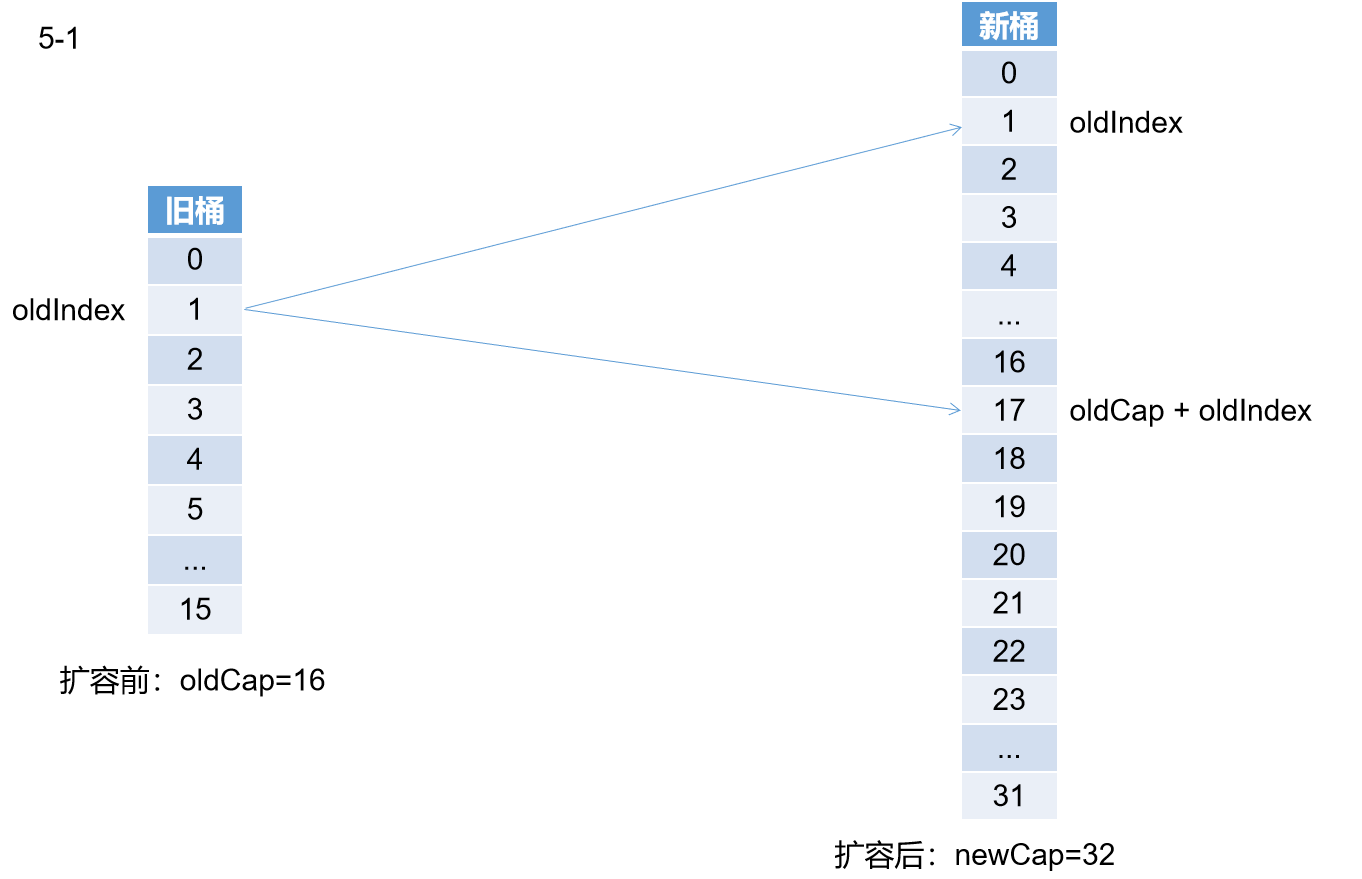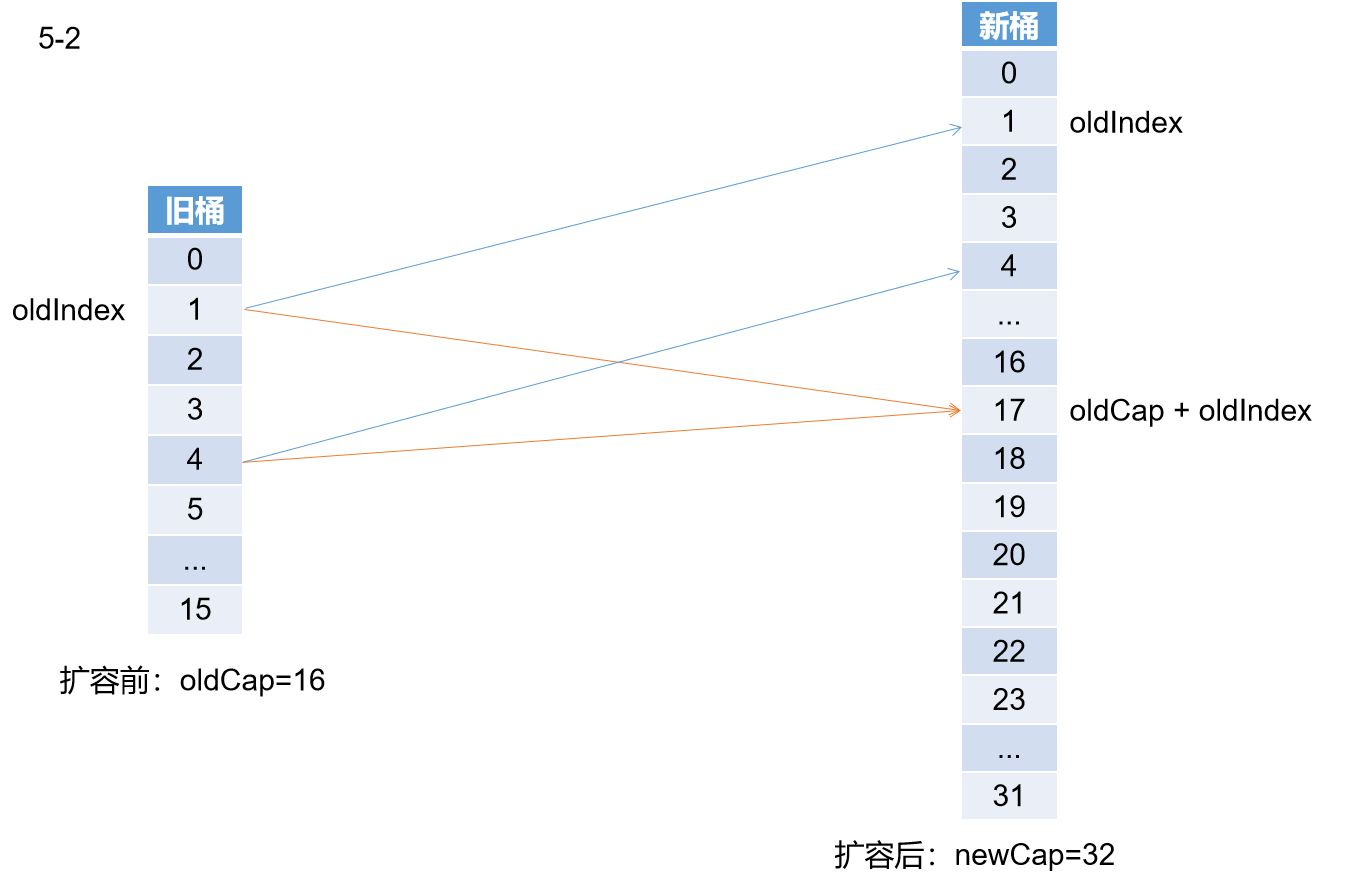5.2.2、数据迁移

//ln新链表，不需要移动的节点重新组组织成的链表。
//hn新链表，需要移动的节点重新组织成的链表
Node<K,V> ln, hn;int runBit = fh & n;

1 for (Node<K,V> p = f.next; p != null; p = p.next) {
2     int b = p.hash & n;
3     if (b != runBit) {//相同跳过
4         runBit = b;
5         lastRun = p;
6     }
7 }

1 if (runBit == 0) {// runBit== 0 表示该节点不需要移动
2     ln = lastRun;
3     hn = null;
4 }
5 else {
6     hn = lastRun;
7     ln = null;
8 }//end

1 for (Node<K,V> p = f; p != lastRun; p = p.next) {
2     int ph = p.hash; K pk = p.key; V pv = p.val;
3     if ((ph & n) == 0)
4         ln = new Node<K,V>(ph, pk, pv, ln);
5     else
6         hn = new Node<K,V>(ph, pk, pv, hn);
7 }上述代码段1迭代找到了lastRun即指向node（11），代码段2将lastRun赋值给hn。代码段3执行过程如下

1，将node(1)拼接到ln
2，将node(3)拼接到hn，此时注意，hn已经lastRun指向的节点node(11)，此时hn=3—>11—>15—>19—>null
3，处理node(5)拼接到ln
4，处理...

1 setTabAt(nextTab, i, ln);
2 setTabAt(nextTab, i + n, hn);
3 setTabAt(tab, i, fwd);
4 advance = true;

ln和hn两个链表各回各家各找各妈。

1 else if ((fh = f.hash) == MOVED)
2     tab = helpTransfer(tab, f);

 1 final Node<K,V>[] helpTransfer(Node<K,V>[] tab, Node<K,V> f) {
2     Node<K,V>[] nextTab; int sc;
3     if (tab != null && (f instanceof ForwardingNode) &&//条件判断
4         (nextTab = ((ForwardingNode<K,V>)f).nextTable) != null) {//从fwd节点中取出新table
5         int rs = resizeStamp(tab.length);
6         while (nextTab == nextTable && table == tab &&
7                (sc = sizeCtl) < 0) {
8             if ((sc >>> RESIZE_STAMP_SHIFT) != rs || sc == rs + 1 ||
9                 sc == rs + MAX_RESIZERS || transferIndex <= 0)
10                 break;
11             if (U.compareAndSwapInt(this, SIZECTL, sc, sc + 1)) {//修改sizeCtl = sizeCtl + 1，表示多了一个线程参与扩容
12                 transfer(tab, nextTab);
13                 break;
14             }
15         }
16         return nextTab;
17     }
18     return table;
19 }

# 六、get过程源码

 1 public V get(Object key) {
2     Node<K,V>[] tab; Node<K,V> e, p; int n, eh; K ek;
4     if ((tab = table) != null && (n = tab.length) > 0 &&
5         (e = tabAt(tab, (n - 1) & h)) != null) {//取桶
6         if ((eh = e.hash) == h) {//key相同直接返回
7             if ((ek = e.key) == key || (ek != null && key.equals(ek)))
8                 return e.val;
9         }
10         else if (eh < 0)//hash < 0 表示正在扩容
11             //在这里需要非常注意的一点，扩容后的桶会放入fwd节点
12             //该节点hash = MOVED，fwd.nextTable为扩容后新的数组。
13             return (p = e.find(h, key)) != null ? p.val : null;
14         while ((e = e.next) != null) {//迭代链表
15             if (e.hash == h &&
16                 ((ek = e.key) == key || (ek != null && key.equals(ek))))
17                 return e.val;
18         }
19     }
20     return null;
21 }

get源码只关注下面这行

return (p = e.find(h, key)) != null ? p.val : null;

static final class ForwardingNode<K,V> extends Node<K,V> {
final Node<K,V>[] nextTable;
ForwardingNode(Node<K,V>[] tab) {
super(MOVED, null, null, null);
this.nextTable = tab;
}
}

 1 Node<K,V> find(int h, Object k) {
2     // loop to avoid arbitrarily deep recursion on forwarding nodes
3     outer: for (Node<K,V>[] tab = nextTable;;) {//迭代nextTable
4         Node<K,V> e; int n;
5         if (k == null || tab == null || (n = tab.length) == 0 ||
6             (e = tabAt(tab, (n - 1) & h)) == null)
7             return null;
8         for (;;) {
9             int eh; K ek;
10             if ((eh = e.hash) == h &&
11                 ((ek = e.key) == k || (ek != null && k.equals(ek))))
12                 return e;
13             if (eh < 0) {
14                 if (e instanceof ForwardingNode) {
15                     tab = ((ForwardingNode<K,V>)e).nextTable;
16                     continue outer;
17                 }
18                 else
19                     return e.find(h, k);
20             }
21             if ((e = e.next) == null)
22                 return null;
23         }
24     }
25 }

OK，很明确了，确实是从nextTable中查找的。

# 七、ConcurrentHashMap并发控制如上图，线程1进行put操作，这时发现size > sizeCtl。开始进行扩容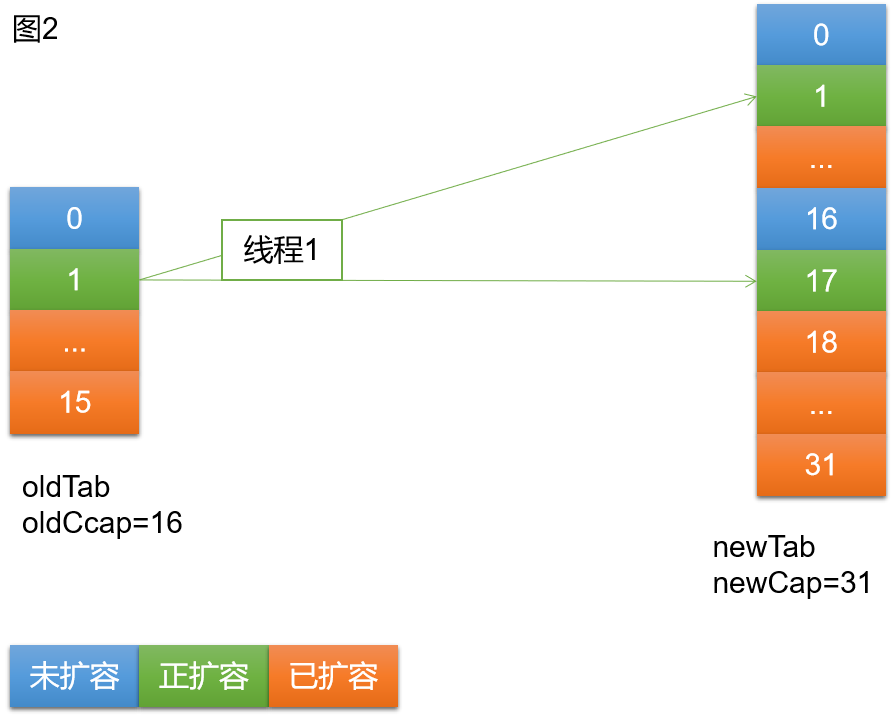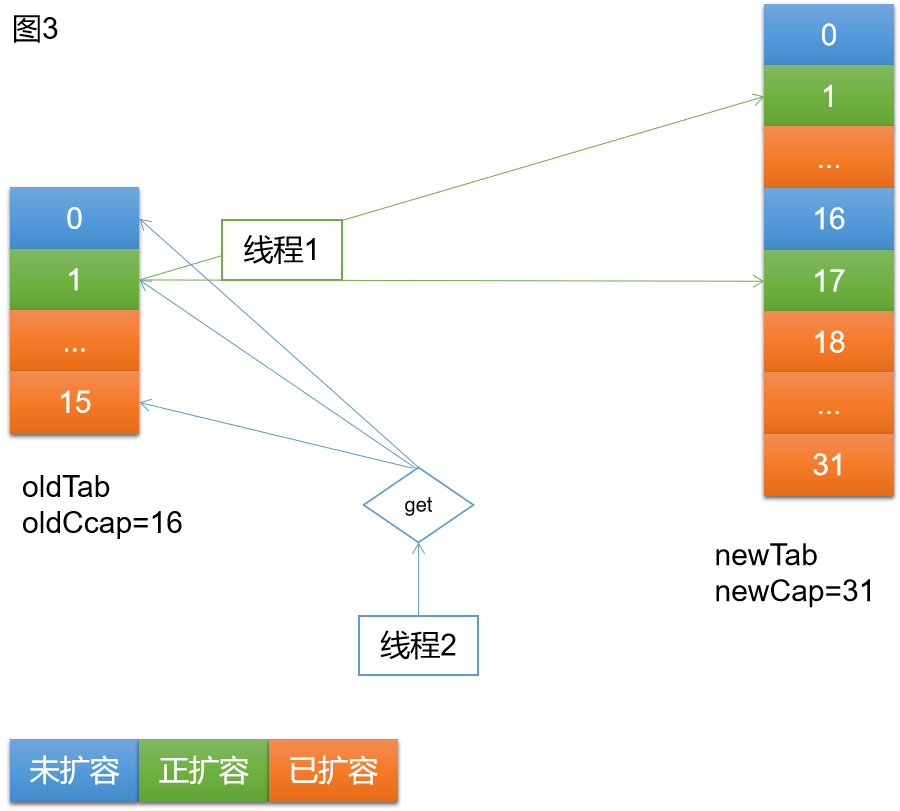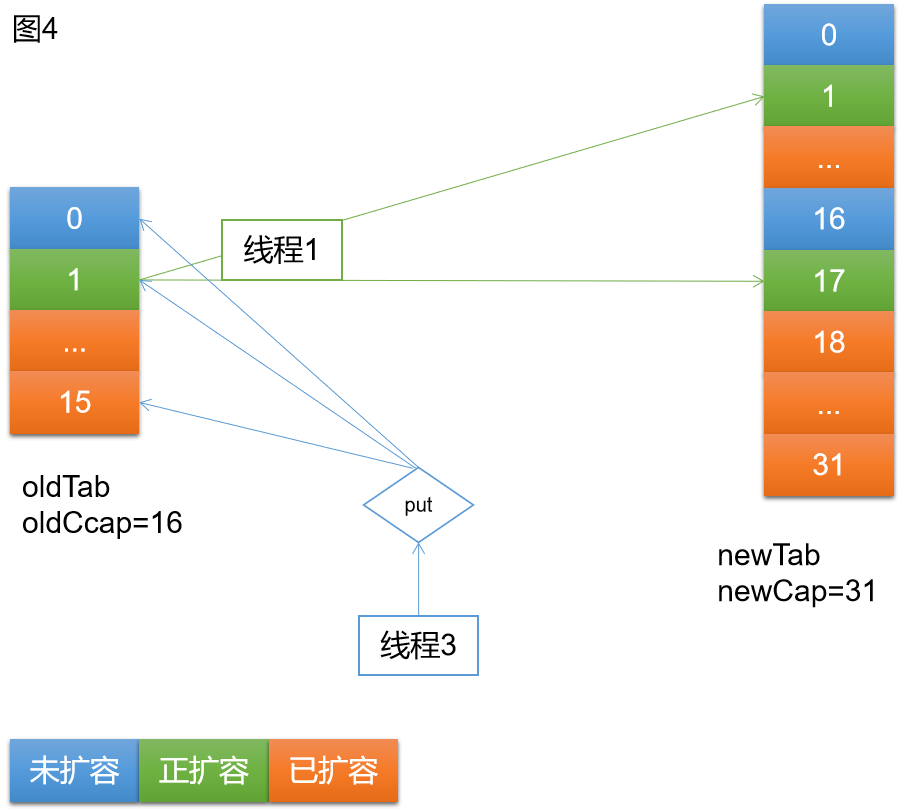如上图4，当线程1进行扩容时，线程3进来执行put，同样存在三种可能的情况

OK，以上就是ConcurrentHashMap关于get put resize的并发控制，从以上过程可见，存在锁竞争的情况很有限，即使存在锁竞争，也是进行自旋，而不会阻塞线程。可见ConcurrentHashMap能做到高效的并发读。

如有错误的地方还请留言指正。
原创不易，转载请注明原文地址：https://www.cnblogs.com/hello-shf/p/12183263.html

posted @ 2020-01-14 16:14  超级小小黑  阅读(...)  评论(...编辑  收藏